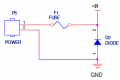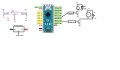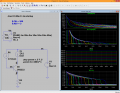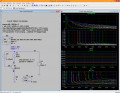# Surge Protection for Circuit

#### bobdxcool

Joined Feb 12, 2012
39
I am building a liquid dispenser system comprised of arduino, dc motor (6V 200mA), 2n2222 transistor, float switch. All these are powered by a regular 5V 1A DC adapter (off the shelf). Do the DC adapters ( made in china) offer surge protection ? If not, how I do implement surge protection ?
I already have a 250V 1amp glass fuse and IN4001 connected (to prevent circuit damage due to reverse polarity connection of adapter) as per the image attached below. (Adapter +ve and -ve connected to P5 screw connector pins 1 and 2).#### ci139

Joined Jul 11, 2016
1,464
http://danyk.cz/impulz4_en.html ... there may be an output Schottky (to enable capacitive loads - then output overdraw will blow it)

considering the 6V 200mA may have a startup current 3x to 6x you may want to use slow blowing exact (read the one that suits the motor and Max supply output) fuse after your current setup . . . or you may want to "buffer"/isolate your motor's power (input) by an input smoothing filter . . . other options are also avilable

#### bobdxcool

Joined Feb 12, 2012
39
http://danyk.cz/impulz4_en.html ... there may be an output Schottky (to enable capacitive loads - then output overdraw will blow it)

considering the 6V 200mA may have a startup current 3x to 6x you may want to use slow blowing exact (read the one that suits the motor and Max supply output) fuse after your current setup . . . or you may want to "buffer"/isolate your motor's power (input) by an input smoothing filter . . . other options are also avilable
Thanks for your reply. I am using a 2N2222A transistor with 180 ohms connected at the base to limit the current to approx 200mA. This should be fine, right? Also, you recommend adding an RC filter across the motor ?
With regards to the surge, I was referring to the kind where there is a surge on the AC line, which would in turn burn out the DC adapter and then the DC circuitry. Is that a possibility?

#### ci139

Joined Jul 11, 2016
1,464
This should be fine, right?
it will do some limiting - but might be not exactly what you want to achieve . . . if its collector load the base resistor should be around
(Vdd - 0.7)/(200mA / ß) . . . if ß is about 200 and Vdd is +5V the base resistor would be 4k3 Ω
Also, you recommend adding an RC filter across the motor ?
(( it's only one possibility to cut motor startup load on the supply ))
series R) from the main (+5V) supply to parallel capacitor with 2N2222 and motor
if your 2N2222 CE drops about 400mV (at about 2.5 ... 3 mA base current Fig.4) the 4k3 above should be about 1k5
the motor !supposedly! has 4.3V/200mA 21.5 Ω impedance
the transistor's Rce ≈ 400mV/200mA = 2Ω (a bit unlikely)
the series R (above)
might be 2 to 3 Ω so it won't let the MOTOR-node to pull over 1A from the supply at startup (the capacitor would provide some startup current , which will not be drawn from the supply at the time the motor starts but at the time the circuit is powered on and that requires limitting the startup inrush current)

which means there is no simple way to set up a protection = you must know how much and for how long your +5V source withstands without getting damaged --e.g.-- as SOA https://en.wikipedia.org/wiki/Safe_operating_area for transistors

#### bobdxcool

Joined Feb 12, 2012
39
it will do some limiting - but might be not exactly what you want to achieve . . . if its collector load the base resistor should be around
(Vdd - 0.7)/(200mA / ß) . . . if ß is about 200 and Vdd is +5V the base resistor would be 4k3 Ω

(( it's only one possibility to cut motor startup load on the supply ))
series R) from the main (+5V) supply to parallel capacitor with 2N2222 and motor
if your 2N2222 CE drops about 400mV (at about 2.5 ... 3 mA base current Fig.4) the 4k3 above should be about 1k5
the motor !supposedly! has 4.3V/200mA 21.5 Ω impedance
the transistor's Rce ≈ 400mV/200mA = 2Ω (a bit unlikely)
the series R (above)
might be 2 to 3 Ω so it won't let the MOTOR-node to pull over 1A from the supply at startup (the capacitor would provide some startup current , which will not be drawn from the supply at the time the motor starts but at the time the circuit is powered on and that requires limitting the startup inrush current)

which means there is no simple way to set up a protection = you must know how much and for how long your +5V source withstands without getting damaged --e.g.-- as SOA https://en.wikipedia.org/wiki/Safe_operating_area for transistors
When transistor operates as a switch(in saturation state), the beta value to be considered is 10 (general thumb of rule), right ?

#### ci139

Joined Jul 11, 2016
1,464
it might be $$\frac{\beta}{2\pi}$$ or other depending also on frequency besides the Ic . . .and the transistor technology
...
for the bipolar constant current sources the most practical value is about "ß=5"

so averaging over choices $$\frac{5+10+\frac{\beta}{6}}3=5+\frac{\beta}{18}$$ ??? who knows . . .

it's Fig3 Fig.4 on the 2N2222 's d/s
as
Fig.3 $$\rightarrow$$ ß(Ic=200mA)≈300/2 . . . e.g. Ib≈200mA/150=1.33mA (@ 25°C)
Fig.4 $$\rightarrow$$ Vce(Ic=200mA,Ib=1.3mA)≈600 ... 700mV . . . . versus $$\rightarrow$$ Vce(Ic=200mA,Ib=3mA)≈200 ... 300mV (@ 25°C)

there is something $V_{CE}=\left(±\right)\frac1{\Lambda}ln\frac{α_I\left[{1-\frac{I_C}{I_B}\frac{\left({1-α_N}\right)}{α_N}}\right]}{\left[{1+\frac{I_C}{I_B}\left({1-α_I}\right)}\right]}$

where $\Lambda=\frac q{\eta KT}\ , \frac1{\Lambda}≈26mV\ @\ 25°C\ , \eta=1\\ ?\ α_N=\frac1{1+\frac1{ß_{FW}}}\\ ?\ α_I=\frac1{1+\frac1{ß_{REV}}}$

Last edited:

#### bobdxcool

Joined Feb 12, 2012
39
I am making a automatic water dispenser (schematics attached) using DC motor, solenoid and IR sensor module (https://robokits.co.in/sensors/ir-a...MIjbWz7Krm6QIVVwQrCh1H9gv2EAQYAyABEgJoCPD_BwE).

Submersible Dc Motor pump: 3-6V DC 100-220mA. Driven by 2NAAAA2 transistor.
Solenoid Valve water: 12V DC(rated 0.6Amps). Driven by TIP120 transistor.
5V IR sensor module connected to input pin of arduino.

Now, I want to make sure that the noise from the motor or solenoid doesn't affect the other components in the circuit. What other components (resistors, capacitors, etc) should I add at different points of the circuit to avoid noise ? Is some sort of RC filter needed to be added to the IR sensor output pin going to the arduino input ?

Also, I am planning to use a bigger 24V DC motor pump (rated 2Amps) in place of existing DC motor pump for another project. What precautions to be taken in this case to avoid noise?

Or is a two channel relay board (probably the ones with optocouplers) a better option without transistors?

And I am planning to add a 250V 2Amp glass fuse for overcurrent protection along with a diode (IN4007) for reverse polarity protection between the power supply adapter and the LM7805 IC.#### ci139

Joined Jul 11, 2016
1,464.
.
.
.
+ seems the above is quite not valid Vce(Ic,Ib,...) formula (from the GE's Transistor manual).
.
.
.
______________
summary ;
according to simulation of the 2N2222 (the forward ß=200) your saturation for 200mA Ic is above Ib1.8mA (Tj=25°C)
according to d/s of the 2N2222 (the forward ß=300) your saturation for 200mA Ic is above Ib3mA (Tj=25°C)
for the 2N2222 i have the ß≈200 ... so the Ib could be above/equal the 4.5mA (Rb.max=950Ω @ 5V Vdd) -- that if the T≈25°C and there is enough voltage head room for the motor to run at it's nominal power . . .

is why you should test everything out at module level in real

other than that . . . the wall adapters (depending on type) may respond to "OUTP overload" differently
• no protection = the voltage drops fast after exceeding the nominal power ((that to unknown extent))
(the supply may survive a short period short circuit ... as well as not)
• protection = depending on protection type -- the supply may : switch off until power is recyled , starts pulsing , folds back the current , ?other

custom MOSFET SC electronic fuse